[Last time](https://forum.azimuthproject.org/discussion/2267/lecture-50-chapter-3-left-kan-extensions/p1) we built new databases from old using a left Kan extension. Let's compare how a right Kan extension works in the exact same situation! Having a good feel for the difference between left and right adjoints, and in particular left and right Kan extensions, is one of the marks of a true category theorist. It takes a while, but the key is thinking about examples.

We saw that the left Kan extension had the ability to automatically extend a database from a small schema like this: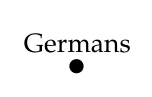to a larger one like this: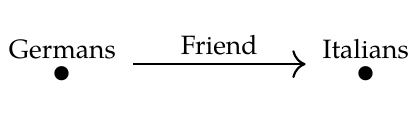This goes 'against the grain'. What's _easy_ to do is restrict a database from a larger schema to a smaller one by discarding information - in this case, all information involving Italians. Going from a smaller schema to a larger one is hard because there's not really enough information. The left Kan extension does this by systematically creating new entries to serve as 'fillers' for missing information. In our example, it makes up a bunch of anonymous Italians to serve as friends for the Germans.

And since the left Kan extension is a left adjoint, it does this in a 'liberal' or 'generous' way. Mathematically, we say it does this in a **free** way. It takes a while to understand what this mean, and it's a bit hard to explain in words: the definition of left Kan extension is the way to make the idea truly precise. But _roughly_ speaking, the left Kan extension freely makes up all the entries that are needed, obeying only the equations that are needed to get a valid database. For example, if you hand Mr. Left Kan Extension this database: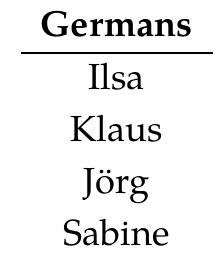it will create this database of Germans, Italians, and which Germans have which Italians as friends: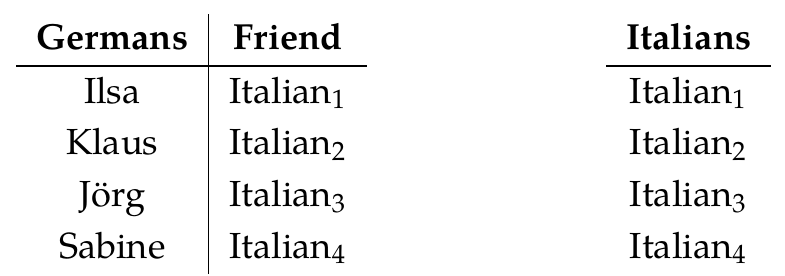So, it makes up only the Italians that are needed for a valid database - one to be a friend of each German - and it doesn't make any of these Italians the same as any other, because that's not required.

The right Kan extension is _another_ systematic way of solving the same problem. Since it's a right adjoint, it does this in a 'conservative' or 'cautious' way. In the same example, it creates this database: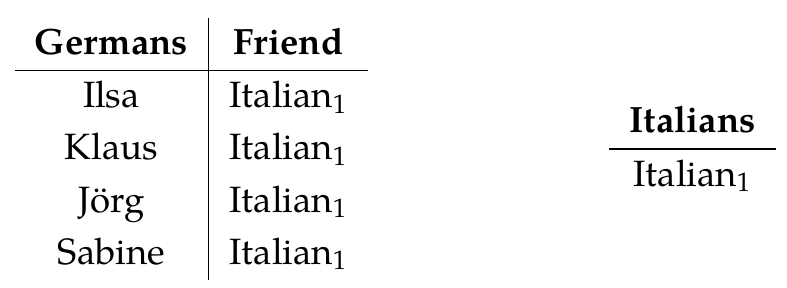This time it makes up just one Italian! In general, the right Kan extension makes up all the entries that are _possible_, but imposes all the equations that are _possible_ in a valid database.

Let's look at the general theory a bit more. As before, we start with two categories \$$\mathcal{C}\$$ and \$$\mathcal{D}\$$, which serve as database schemas. Then we choose a functor \$$G: \mathcal{D} \to \mathcal{C}\$$. This gives a process for turning any database built using the first schema, say

$F: \mathcal{C} \to \mathbf{Set}$

into a database built using the second schema, namely

$F \circ G : \mathcal{D} \to \mathbf{Set} .$

This process is actually a functor:

$\text{composition with } G : \mathbf{Set}^\mathcal{C} \to \mathbf{Set}^\mathcal{D} .$

The left and right Kan extensions are two ways to _reverse_ this process: they are the left and right adjoints of the above functor!

Today we're looking at the right Kan extension. This is defined as follows: there's a natural one-to-one correspondence between

$\textrm{natural transformations } \alpha: F \circ G \Rightarrow H$

and

$\textrm{natural transformations } \beta: F \Rightarrow \textrm{Ran}_G(H) .$

Let's see how it works in our example. We're taking \$$\mathcal{D}\$$ to be the free category on this graphand \$$\mathcal{C}\$$ to be the free category on this bigger graph:We're taking \$$G : \mathcal{D} \to \mathcal{C} \$$ to be the obvious inclusion of \$$\mathcal{D}\$$ in the bigger category \$$\mathcal{C}\$$.

If we take \$$F : \mathcal{C} \to \mathbf{Set} \$$ to be this database: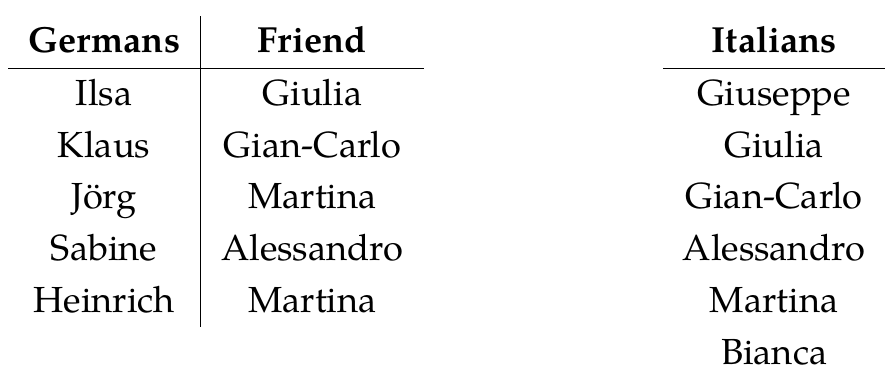then \$$F \circ G\$$ is this database: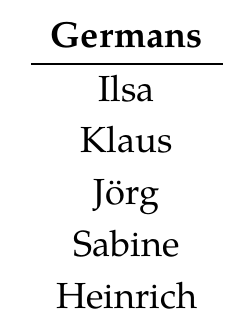So, it's just the restriction of \$$F\$$ to the smaller category \$$\mathcal{D}\$$.

But suppose we want to reverse this process! We start with a functor defined on the smaller category, say \$$H : \mathcal{D} \to \mathbf{Set}\$$. This is a database built on our smaller schema:Then we extend it to the larger category using a right Kan extension, getting a functor \$$\textrm{Ran}_G(H): \mathcal{C} \to \mathbf{Set}\$$. This gives a database built on our larger schema, and I claim it looks like this:Can you check this? It's just a matter of patiently unraveling all the definitions! I won't make you prove that \$$\textrm{Ran}_G(H)\$$ really meet all the conditions of a right Kan extension, just that it seems to be on the right track.

**Puzzle 160.** We know from the definition of right Kan extension that there should be a natural one-to-one correspondence between

$\textrm{natural transformations } \alpha: F \circ G \Rightarrow H$

and

$\textrm{natural transformations } \beta: F \Rightarrow \textrm{Ran}_G(H) .$

Can you guess how it works for our particular choice of \$$F,G\$$ and \$$H\$$? By counting the number of natural transformations of the two kinds you can show that there _exists_ a one-to-one correspondence between them, but there should be an 'obvious best' one-to-one correspondence.

**[To read other lectures go here.](http://www.azimuthproject.org/azimuth/show/Applied+Category+Theory#Chapter_3)**